ExetoolsFind the Algorithm
 Register Forum Rules FAQ Calendar Mark Forums Read

 Notices HTTP connection will be closed soon. Please visit eXeTools with HTTPS in the future. https://forum.exetools.com This is the ONLY ONE domain that we use. Follow @exetools on Twitter and send me a message, I will choose whether to send the invitation code. Any password problems please mailto: 883600(at)qq(dot)com

#1
 mcr4ckFriend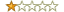Join Date: Nov 2019 Posts: 14 Rept. Given: 0 Rept. Rcvd 1 Time in 1 Post Thanks Given: 12 Thanks Rcvd at 1 Time in 1 Post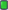Find the Algorithm

I would like to see some of my friends take the algorithm out of these formulas
They all count on one algorithm

0101=70f5
de50=2c8c
0102=dde2
ffff-5967
#2
 chantsFamily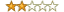Join Date: Jul 2016 Posts: 469 Rept. Given: 2 Rept. Rcvd 33 Times in 19 Posts Thanks Given: 394 Thanks Rcvd at 745 Times in 342 PostsAn infinite number of mathematical or algorithmic mappings are possible. Probably you need to be more specific on what operations are allowed and maximum number of ops as well as maybe more values. Otherwise easy enough:
(x&0xf==1)*0x70f5+(x&0xf==0)*0x2c8c+(x&0xf==2)*0xdde2+(x&0xf==0xf)*0x5967

Technically speaking that one liner is an algorithm upon which they all rely. I'm not sure how to properly mathematically phrase what I feel you ar asking, as doubtlessly not my silly example, but it's interesting in how hard the question might be to exactly specify. I would think something like the minimal kolmogorov complexity but even then maybe this formula is simpler with a mere 4 cases. I would like to see not only the intended answer but how are we to ask in a way which excludes all the endless wrong onesThe Following User Says Thank You to chants For This Useful Post: mcr4ck (01-14-2020)
#3
 mcr4ckFriendJoin Date: Nov 2019 Posts: 14 Rept. Given: 0 Rept. Rcvd 1 Time in 1 Post Thanks Given: 12 Thanks Rcvd at 1 Time in 1 PostI didn't understand anything from the formula
I think it's flawed
On the other hand, the numbers that are written together are calculated by a formula
That is, they are separate
0101=70f5
or
de50=2c8c
or
0102=dde2
or
ffff=5967

Here's the formula for calculating this
That is to say, if we give an input to these numbers, give me an output according to the numbers above.

for example:

in 0101 ==> out 70f5
or

in de50 ==> out 2c8c

or
in 0102 ==> out dde2
#4
 chantsFamilyJoin Date: Jul 2016 Posts: 469 Rept. Given: 2 Rept. Rcvd 33 Times in 19 Posts Thanks Given: 394 Thanks Rcvd at 745 Times in 342 PostsMy formula used bit wise and &, equality comparison ==, implicit cast of true/false to 1/0 and integer multiplication *, and integer addition +. It should work in C or Javascript or what have you. x is the input, the result is the output. Exactly as you specify it.

This works since the last digits are all unique. By bitwise and with 0xf, we get the last digit of the input and compare with each unique last digit. If equal it will result in 1 times the correct output value otherwise 0. Then summed all together maps them properly.

Any one to one mapping can be done without the bitwise and, using only the other 4 operations. Of course it will only work for individual inputs then and will always be zero for not yet seen numbers. Currently it works for individual input last digits that match and is 0 for all other inputs.

Last edited by chants; 01-14-2020 at 04:44.
 The Following User Says Thank You to chants For This Useful Post: mcr4ck (01-14-2020)
#5
 mcr4ckFriendJoin Date: Nov 2019 Posts: 14 Rept. Given: 0 Rept. Rcvd 1 Time in 1 Post Thanks Given: 12 Thanks Rcvd at 1 Time in 1 PostI didn't really understand what you meant
Can you give an example
It means giving in and taking out
#6
 chantsFamilyJoin Date: Jul 2016 Posts: 469 Rept. Given: 2 Rept. Rcvd 33 Times in 19 Posts Thanks Given: 394 Thanks Rcvd at 745 Times in 342 PostsJust open a Python 3.7 console:
Code:
```>>> x=0x0101
>>> hex((x&0xf==1)*0x70f5+(x&0xf==0)*0x2c8c+(x&0xf==2)*0xdde2+(x&0xf==0xf)*0x5967)
'0x70f5'
>>> x=0xde50
>>> hex((x&0xf==1)*0x70f5+(x&0xf==0)*0x2c8c+(x&0xf==2)*0xdde2+(x&0xf==0xf)*0x5967)
'0x2c8c'
>>> x=0x0102
>>> hex((x&0xf==1)*0x70f5+(x&0xf==0)*0x2c8c+(x&0xf==2)*0xdde2+(x&0xf==0xf)*0x5967)
'0xdde2'
>>> x=0xffff
>>> hex((x&0xf==1)*0x70f5+(x&0xf==0)*0x2c8c+(x&0xf==2)*0xdde2+(x&0xf==0xf)*0x5967)
'0x5967'```
The formula is
Code:
`f(x)=(x&0xf==1)*0x70f5+(x&0xf==0)*0x2c8c+(x&0xf==2)*0xdde2+(x&0xf==0xf)*0x5967`
You should learn how and why this works and try to understand the basic code. Its not the solution you are looking for but simply asking for a mapping function with a few points is pretty generic. Without having some white box information or a lot of values or some obvious pattern, my answer could be as good as any.
 The Following 2 Users Say Thank You to chants For This Useful Post: Abaddon (01-19-2020), mcr4ck (01-18-2020)
#7
 mcr4ckFriendJoin Date: Nov 2019 Posts: 14 Rept. Given: 0 Rept. Rcvd 1 Time in 1 Post Thanks Given: 12 Thanks Rcvd at 1 Time in 1 PostToo many codes I just cited, for example
You should not relate to each other
Because I want to get the output
Now if I change the input the output will change too
I want a single formula that matches the input and output I gave
That means the number of entries is too high, and I only said eight
You have included all four outputs in the calculation

in 0202===> out ?
#8
 Abaddon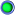FriendJoin Date: May 2016 Posts: 25 Rept. Given: 0 Rept. Rcvd 1 Time in 1 Post Thanks Given: 57 Thanks Rcvd at 24 Times in 14 PostsWhat chants is saying, is that you can't derive the algorithm from 4 challenge-response combinations.
Even if you had a lot more combinations of challenge-response codes, it would probably be difficult to deduce the formula.

The formula chants provided works with the four combinations you provided. It does not mean that it is the formula that originally gave them. This means that if you had a fifth pair of challenge-response codes, the output derived from chant's formula would almost certainly mismatch the responce code of your black-box.
 The Following User Says Thank You to Abaddon For This Useful Post: chants (01-20-2020)
#9
 mcr4ckFriendJoin Date: Nov 2019 Posts: 14 Rept. Given: 0 Rept. Rcvd 1 Time in 1 Post Thanks Given: 12 Thanks Rcvd at 1 Time in 1 Posthi

for more example:

3300 -> 729C
3301 -> BEF5
3302 -> 4BE2
3303 -> 111B
3304 -> 2750
3305 -> 7DA9
3306 -> 21A6
3307 -> AEDF
3308 -> E594
3309 -> 77ED
330A -> 805A
330B -> 4993
330C -> A148
330D -> D121
330E -> B6DE
330F -> 31D7
3310 -> 2DCC
3311 -> 5FA5
3312 -> 6352
3313 -> F40B
3315 -> B999
3316 -> C356
3317 -> 610F
3318 -> 2804
3319 -> 5A9D
331A -> 348A
331B -> 9403
331C -> 6DF8
331D -> 9851

Can we make an algorithm for these?
#10
 mcr4ckFriendJoin Date: Nov 2019 Posts: 14 Rept. Given: 0 Rept. Rcvd 1 Time in 1 Post Thanks Given: 12 Thanks Rcvd at 1 Time in 1 Posthi

for more example:

3300 -> 729C
3301 -> BEF5
3302 -> 4BE2
3303 -> 111B
3304 -> 2750
3305 -> 7DA9
3306 -> 21A6
3307 -> AEDF
3308 -> E594
3309 -> 77ED
330A -> 805A
330B -> 4993
330C -> A148
330D -> D121
330E -> B6DE
330F -> 31D7
3310 -> 2DCC
3311 -> 5FA5
3312 -> 6352
3313 -> F40B
3315 -> B999
3316 -> C356
3317 -> 610F
3318 -> 2804
3319 -> 5A9D
331A -> 348A
331B -> 9403
331C -> 6DF8
331D -> 9851

Can we make an algorithm for these?
#11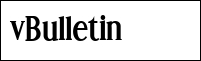niculaitaFamilyJoin Date: Jun 2011 Location: here Posts: 1,127 Rept. Given: 855 Rept. Rcvd 85 Times in 57 Posts Thanks Given: 2,640 Thanks Rcvd at 423 Times in 302 Postsyes, obligate/set anytine 331D as enter values and you will know anytime the answer__________________
Decode and Conquer

 Thread ToolsShow Printable VersionEmail this Page Display ModesLinear ModeSwitch to Hybrid ModeSwitch to Threaded ModePosting Rules You may not post new threads You may not post replies You may not post attachments You may not edit your posts BB code is On Smilies are On [IMG] code is Off HTML code is Off Forum Rules

All times are GMT +8. The time now is 23:18.

 Aaron's homepage - TopAlways Your Best Friend: Aaron, JMI, ahmadmansoor, ZeNiX
( 1998 - 2020 )